Capacitor Guide

# The temperature characteristics of electrostatic capacitance

Greetings everyone.
This technical column describes the basic facts about capacitors.
This lesson describes the temperature characteristics of electrostatic capacitance.

## Temperature characteristics

### 1. Temperature characteristics of various capacitor types

The electrostatic capacitance of capacitors generally varies according to the operating temperature. Capacitors are said to have good temperature characteristics when this variance width is small or poor temperature characteristics when the variance width is large. When using capacitors in locations with high operating temperatures such as inside automobile engine rooms, or in electronic equipment used in cold regions such as the Antarctic, the design must take into account the operating environment conditions.

Figure 1 shows the capacitance change rate vs. temperature characteristics of various capacitor types with typical temperature characteristics.

Conductive polymer aluminum electrolytic capacitors (Polymer Al), film capacitors (Film), and temperature-compensating-type multilayer ceramic capacitors (MLCC<C0G>) are shown as examples of capacitors with good temperature characteristics. In contrast, conductive polymer tantalum electrolytic capacitors (Polymer Ta) and high dielectric constant-type multilayer ceramic capacitors (MLCC<X5R, Y5V>) exhibit large changes in capacitance at high temperatures.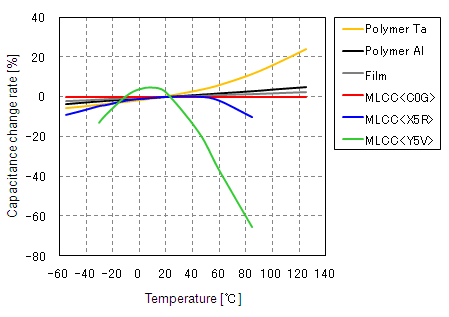Figure 1. Capacitance change rate vs. temperature characteristics of various capacitor types (Example)

### 2. Temperature characteristics of multilayer ceramic capacitors

Multilayer ceramic capacitors are broadly divided into two types, and the temperature characteristics differ according to the type.
(1) One type is temperature-compensating-type multilayer ceramic capacitors, which are categorized as Class 1 in the official standards. This type uses dielectric materials such as titanium oxide or calcium zirconate, and the electrostatic capacitance changes in a fairly linear manner with respect to temperature. The slope with respect to temperature is called the temperature coefficient, and this value is expressed in units of 1/1,000,000 per 1°C [ppm/°C]. The temperature coefficient is defined by Equation 1 below based on the capacitance value C25 at the reference temperature (this is 20°C in the IEC and JIS standards, and 25°C in the EIA standard, but 25°C is used as the reference here) and the capacitance value CT at the category upper limit temperature (maximum operating temperature: maximum design ambient temperature at which the capacitor can be continuously used).

The EIA standard specifies various temperature coefficients of electrostatic capacitance within the range of 0 ppm/°C to -750 ppm/°C. Figure 2 below shows the temperature characteristics of typical materials, and Table 1 shows excerpts of the applied JIS and EIA standards.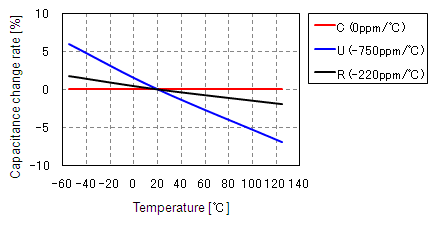Figure 2. Capacitance change rate vs. temperature characteristics of temperature-compensating-type ceramic capacitors (Example)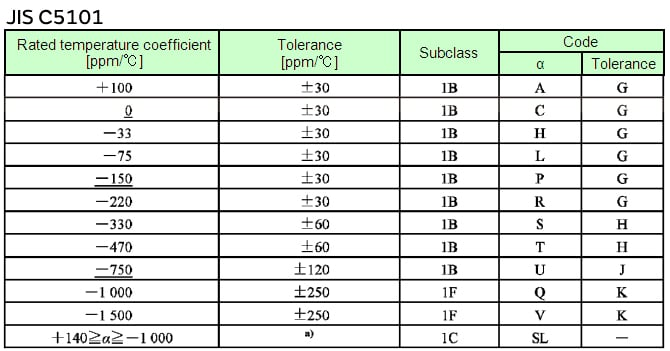Table 1. Temperature coefficient values and tolerance of temperature-compensating-type multilayer ceramic capacitors, and corresponding codes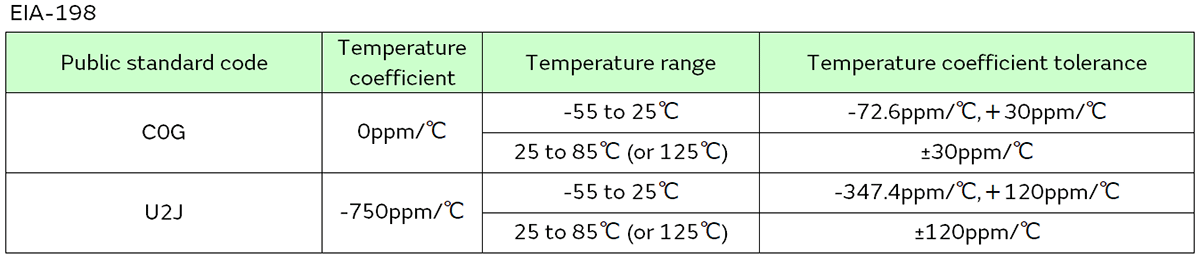Temperature-compensating-type multilayer ceramic capacitors have a small temperature coefficient of electrostatic capacitance (max. ±30 ppm/°C (25°C reference)) within the temperature range of -55°C to +125°C; that is to say, these are C0G characteristics products with a small temperature variance width. However, temperature-compensating-type multilayer ceramic capacitors use dielectric materials with a small dielectric constant, so there is also the demerit that large-capacity products cannot be realized.

(2) The other type is high dielectric constant-type multilayer ceramic capacitors, which are categorized as Class 2 in the official standards. This type uses barium titanate as the dielectric material, and the capacitance value exhibits irregular variance with respect to temperature. For this reason, the electrostatic capacitance vs. temperature characteristic standard values are specified by the maximum and minimum values of the capacitance change rate within the applied temperature range, relative to the capacitance value C25 at the reference temperature (25°C is used as the reference here). (See Equation 2.)

Table 2 shows excerpts of the applied JIS and EIA standard values. There are multiple types of standards, from one that limits the capacitance change rate within the category temperature range to within ±15% (X5R characteristics products), to one that allows capacitance change rates ranging from +22% to -82% (Y5V characteristics products). Recently, X5R characteristics products with a capacitance change rate level of ±15% are accounting for an increasing percentage of capacitors used, which suggests that circuit designers are paying attention to capacitor selection.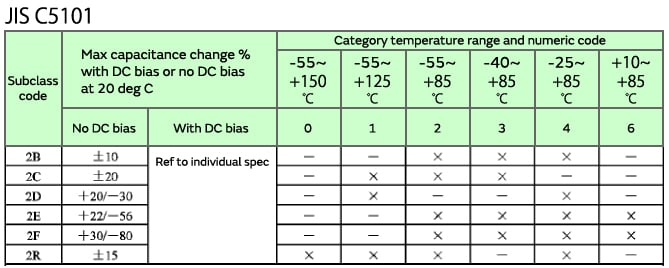Table 2. Temperature characteristic standards of high dielectric constant-type multilayer ceramic capacitors, and corresponding codes